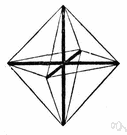# affine transformation

Also found in: Thesaurus, Encyclopedia, Wikipedia.
ThesaurusAntonymsRelated WordsSynonymsLegend:
 Noun 1affine transformation - (mathematics) a transformation that is a combination of single transformations such as translation or rotation or reflection on an axismath, mathematics, maths - a science (or group of related sciences) dealing with the logic of quantity and shape and arrangementtransformation - (mathematics) a function that changes the position or direction of the axes of a coordinate system
Based on WordNet 3.0, Farlex clipart collection. © 2003-2012 Princeton University, Farlex Inc.
References in periodicals archive ?
For analysis, head motion correction is done, the functional images are co-registered to structural images using a six-parameter rigid-body transformation and a 12-parameter affine transformation (8).
Rotation is a case of an affine transformation, and it is widely used in image processing (for example image registration).
* Scale Invariance: If T:[R.sup.2] [right arrow] [R.sup.2] represents a positive affine transformation, that is, T(x, y) = ([r.sub.1]x + [s.sub.1], [r.sub.2]y + [s.sub.2]) for some positive constant [r.sub.i] and some constant [s.sub.i], then we have g([c.sub.1]; [c.sub.2], E, T([u.sub.1], [u.sub.2])) = g([c.sub.1], [c.sub.2], E, [u.sub.1], [u.sub.2]) for any ([c.sub.1], [c.sub.2], E, [u.sub.1], [u.sub.2]) [member of] C.
The aim of coarse registration is to find an affine transformation matrix such that the two body-fitted coordinate systems can be aligned after the affine transformation.
Thereby we use the affine transformation of van der Pauw  in order to replace the original device having anisotropic resistivity by an equivalent device having isotropic conductivity.
The procedure used for the preprocessing comprised the following steps: (1) conversion of RGB images to grayscale, (2) affine transformation, (3) extraction of the region of interest (ROI), and (4) histogram equalization.
As an affine transformation is a nonsingular linear transformation followed by a translation, we regard the mapping relationship between two images as an affine transformation.
The construction of Rainbow includes affine transformation [L.sub.1], central map transformation F, and affine transformation [L.sub.2]; that is,
In the first step, reference image and target image were globally aligned using affine transformation. Region of interest was selected and a mesh of control points was initialized over the region using the affine transformation.
Thus, the affine transformation is optimized with respect to translation, rotation, scaling, and shearing with a mixture of 12 parameters.
Generally, ICP could be adopted on the input feature point clouds to calculate the parameters of an affine transformation matrix.

Site: Follow: Share:
Open / Close